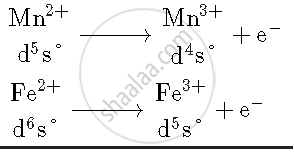Share

# Give Reasons: E° Value for the Mn3+/Mn2+ Couple is Much More Positive than that for Fe3+/Fe2+. - Chemistry

#### Question

Give reasons: E° value for the Mn3+/Mn2+ couple is much more positive than that for Fe3+/Fe2+.

#### Solution

E_(Mn^(3+)"/"Mn^(2+))^@ = more positive

E_(Fe^(3+)"/"Fe^(2+))^@ = less positive

Mn^(2+) = d^5s^@

Mn^(3+) = d^4]

Because Mn2+ is more stable than Mn3+. Hence, Mn3+ will have tendency to go in +2 state.
Whereas in case of Fe, Fe3+ is more stable than Fe2+ hence, it have less positive value.

Is there an error in this question or solution?